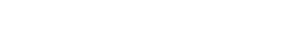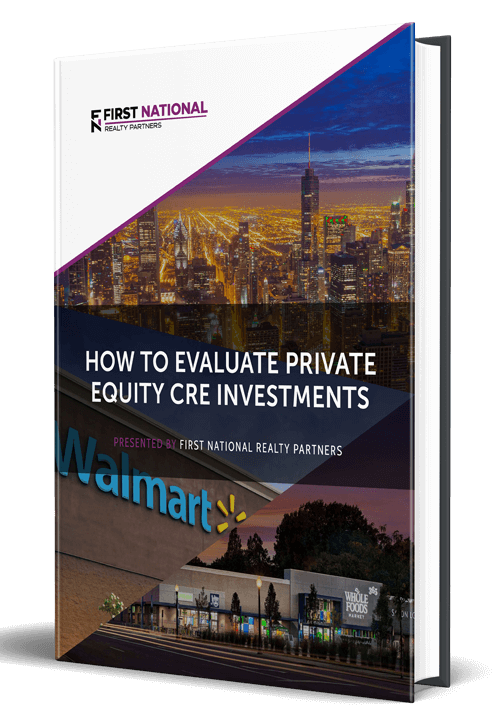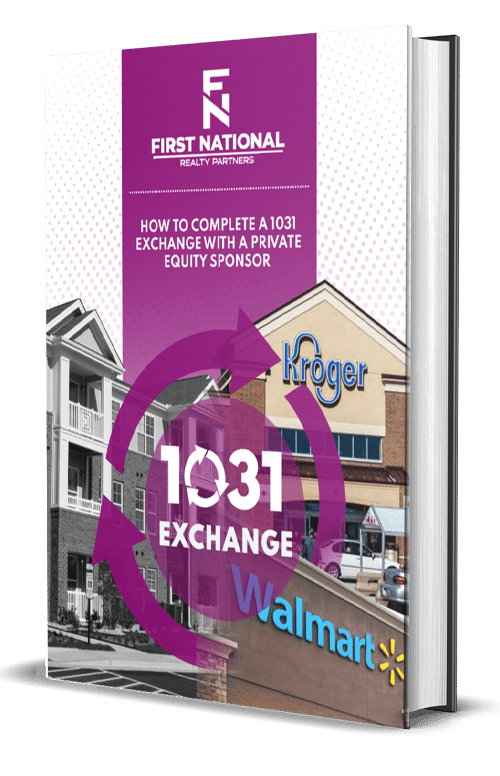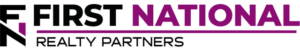# Cap Rate vs. ROI: A CRE Investor’s Guide by FNRP

### Key Takeaways

• The cap rate is a real estate return metric that describes the relationship between a property’s net operating income and its current market value.  It is calculated as net operating income divided by value.
• Return on Investment – ROI – is another real estate return metric that represents a property’s annual return on cash invested.  It is calculated as annual cash return divided by total cash invested.
• The key difference between the cap rate and ROI is debt.  Cap rate assumes that a property is purchased with cash.  ROI assumes that debt is used in the transaction.  If the property was purchased with cash, the cap rate and ROI would be the same.
• Real estate investors can use cap rate as a filter to identify deals that meet their return criteria.  They can also use it to estimate a property’s terminal value based on sales of similar properties.
• ROI can also be used as a way to filter through deals based on return criteria, but its most useful function is to help size the property amount of debt in a deal.
• A good cap rate or a good ROI is relative to an investor’s return criteria.

When an individual or firm is considering a potential commercial real estate investment, there are two questions that are likely at the forefront of their mind:  what is my potential investment return and how will my return be impacted when debt is factored into the equation?  Fortunately, there are commonly used commercial real estate performance metrics that can provide answers to both of these.  They are known as cap rate and ROI.

In this article, we are going to define the cap rate and ROI, how they are calculated, and why they are important in commercial real estate.  By the end, readers should have the information needed to calculate these metrics on their own as part of the pre-purchase due diligence process.

At First National Realty Partners, potential deals must meet certain cap rate and ROI hurdles before we will even consider presenting them to investors.  To learn more about our current investment opportunities, click here.

## What is The Cap Rate?

The capitalization rate<– cap rate for short – is a commercial real estate metric that describes the relationship between a property’s net operating income (NOI) and its market value.It reveals a few key pieces of information about a potential investment property:

• Potential Return:  The cap rate represents the potential rate of return on a property assuming that it was purchased with cash
• Risk:  Because it measures the return, it can also be used as a proxy for the market’s perception of risk.  A higher cap rate means that the market sees more risk and demands higher returns.  A lower cap rate means that the market sees lower risk so they are willing to accept a lower return.
• Market Value:  Based on the inputs in the cap rate calculation, it can also be used to calculate a property’s potential value.  This is particularly helpful when trying to estimate purchase and sales prices in a pro forma.

Given the points above, it can be seen that the cap rate is an important and versatile metric so it stands to reason that commercial real estate investors should be familiar with how it is calculated.

### Calculating the Cap Rate

The cap rate formula is:

Cap Rate = Net Operating Income / Property Value

While the formula itself is straightforward, obtaining the required inputs can be a little bit tricky.

Net Operating Income is calculated as a property’s gross annual income less its operating expenses (property taxes, maintenance, etc) .  Property value isn’t always known at the time the calculation is being made so it could be represented by a value estimate, purchase price, or appraised value.  For example, if a property has NOI of \$100,000 and a value of \$1,000,000, the resulting cap rate is 10%.

## What is ROI?

ROI is an acronym for “Return on Investment” and it represents the annual return that a real estate investor could expect on the purchase of a rental property.

### Calculating ROI

The formula used to calculate ROI is:

Return on Investment = Annual Cash Return / Total Cash Invested

For example, suppose a property had an annual cash return of \$100,000 on a total cash investment of \$1,000,000.  The resulting ROI is 10%.  This description may sound very similar to the cap rate, but a closer look at the formulas for both metrics reveals a key difference.

## Differences Between Cap Rate and NOI

To recap, the following table compares the cap rate and ROI formulas side by side:

 Cap Rate ROI Numerator: Net Operating Income Annual Cash Return Denominator: Property Value Total Cash Investment

There are key differences in these formulas in both the numerator and denominator.  In the numerator, the cap rate uses ROI, which is a before debt service metric, and ROI uses annual cash return, which is net of mortgage payments.  In the denominator, cap rate uses the property value while ROI uses the cash investment.

The key point here is debt.  Cap rate assumes that real estate is purchased with cash, ROI incorporates the impact of debt.  To illustrate the difference, consider a property with the following cash flows:

Gross Income:  \$1,000,000

Operating Expenses:  \$500,000

Annual Debt Service:  \$250,000

Property Value:  \$7,500,000

Total Cash Investment (Down Payment):  \$1,500,000

With these inputs the cap rate and ROI calculations are summarized in the following table:

 Cap Rate ROI Numerator: \$500,000 \$250,000 Denominator: \$7,500,000 \$1,500,000 Result: 6.67% 16.67%

When debt is used in a transaction, the amount of cash injected into the deal is smaller, which makes the return higher in most cases.  Or, put another way, if a property was purchased with cash, the cap rate and ROI would be the same.  But, with debt, there is a difference.  For this reason, there are scenarios in which both of them are useful.

## When to Use The Cap Rate vs. When to Use ROI

### When to Use the Cap Rate

In real estate investing, the cap rate is most useful at two points in the transaction, the beginning and the end.

At the beginning of the transaction, the cap rate is useful as a tool to screen out potential deals that don’t meet an investor’s return criteria.  For example, if an investor requires a return of at least 8%, they would exclude any deals from their search that have a cap rate less than this.

At the end of the transaction, the cap rate is helpful to estimate a property’s “terminal value.”  In a proforma, a real estate investor must project a property’s income and expenses over a multi-year holding period.  At the end of that holding period, NOI can be divided by the cap rate to estimate the sales price of the property.  This sales price is an important input into total return calculations like Internal Rate of Return (IRR) and Net Present Value (NPV).

### When to Use ROI

ROI is also useful as a way to screen potential commercial real estate deals based on their return potential.  But, its biggest utility is as a way to measure the impact of debt on a property.  Specifically, it can help “size” the amount of debt needed to meet certain return hurdles.  If that amount of debt is more than a lender is willing to provide, it may be an indication that there is too much leverage in the deal at a given purchase price.

## So, What is a Good Cap Rate and ROI?

Like most things in real estate, the answer to this question is relative.  There is no objectively “good” cap rate or ROI number.  Good is measured relative to an investor’s risk tolerance and return criteria.  For example, a 10% annual ROI seems great, but if an investor requires 15%, it isn’t good enough.  Same goes for the cap rate.  If an investor wants a cap rate of 8%, they may not settle for 6%.

Individuals should establish their own investment strategy for the cap rate and ROI and use these parameters as key inputs into their ultimate investment decision.

## Summary & Conclusion

The cap rate is a return metric that describes the relationship between a property’s net operating income and its current market value.  It is calculated as net operating income divided by value.

Return on Investment – ROI – is another real estate return metric that represents a property’s annual return on cash invested.  It is calculated as annual cash return divided by total cash invested.

The key difference between the cap rate and ROI is debt.  Cap rate assumes that real estate is purchased with cash.  ROI assumes that debt is used in the transaction.  If the property was purchased with cash, the cap rate and ROI would be the same.

Real estate investors can use cap rate as a filter to identify deals that meet their return criteria.  They can also use it to estimate a property’s terminal value based on sales of similar properties.

ROI can also be used as a way to filter through deals based on return criteria, but its most useful function is to help size the property amount of debt in a deal.

A good cap rate or a good ROI is relative to an investor’s return criteria.

## Interested In Learning More?

First National Realty Partners is one of the country’s leading private equity commercial real estate investment firms. With an intentional focus on finding world-class, multi-tenanted assets well below intrinsic value, we seek to create superior long-term, risk-adjusted returns for our investors while creating strong economic assets for the communities we invest in.

Get instant access to all of our current and past commercial real estate deals.## Subscribe Now

Get the latest news on real estate

Operations and Management

### October 9, 2023

FNRP Spotlight Series

1031 Exchange

## Get More From FNRP## How to Evaluate Private Equity CRE Investments## How to Complete a 1031 Exchange with a Private Equity Sponsor